# JavaScript中的浅拷贝与深拷贝

JS中有两种数据类型，值类型和引用类型，当我们需要把一个变量赋给另一个变量时，对于值类型很简单：

``````let a = 1;
let b = a;
b = 10;
console.log(a, b); // 1, 10
``````

``````let a = {value: 1};
let b = a;
b.value = 10;
console.log(a.value, b.value); // 10, 10
``````

## 浅拷贝

``````let target = {
name: 'John',
age: 20,
friend: {
name: 'Michel',
age: 30
}
}
``````

``````const shallowCopy = (obj) => {
// 判断参数是数组还是对象
const result = Array.isArray(obj) ? [] : {};
for(let key in obj) {
// 使用hasOwnProperty来判断是否是自身属性
// 只拷贝自身属性，不拷贝原型链上的属性，即继承属性
if(obj.hasOwnProperty(key)){
result[key] = obj[key];
}
}

return result;
}
``````

``````let newObj = shallowCopy(target);
newObj.age = 50;
console.log(target.age, newObj.age); //20, 50
``````

``````newObj.friend.age = 50;
console.log(target.friend.age, newObj.friend.age); //50, 50
``````

``````let newObj = Object.assign({}, target); // 这是一层的浅拷贝
let newObj = {...target};  // 这也是一层的浅拷贝
``````

## 深拷贝

### JSON

``````let newObj = JSON.parse(JSON.stringify(target));

newObj.friend.age = 50;
console.log(target.friend.age, newObj.friend.age); //30, 50
``````

``````let target2 = {
name: 'John',
age: 20,
drive: () => {},
girlFriend: undefined
}

let newObj = JSON.parse(JSON.stringify(target2));
console.log(newObj);
``````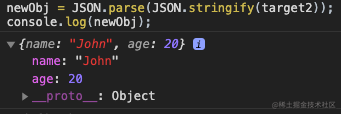### 递归遍历

``````const deepCopy = (obj) => {
const result = Array.isArray(obj) ? [] : {};
for(let key in obj) {
if(obj.hasOwnProperty(key)){
// 如果属性也是对象，递归调用自身
if(obj[key] && typeof obj[key] === 'object'){
result[key] = deepCopy(obj[key])
} else {
result[key] = obj[key];
}
}
}

return result;
}
``````

``````let newObj = deepCopy(target2);
console.log(newObj);
``````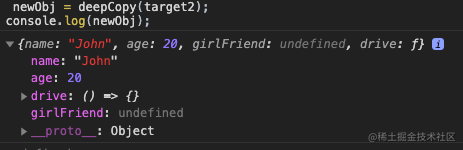### 拷贝Symbol

``````let target3 = {
[Symbol('name')]: 'John',
age: 20,
drive: () => {},
girlFriend: undefined
}
``````

``````let newObj = deepCopy(target3);
console.log(newObj);
``````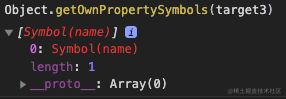`Reflect.ownKeys`会返回对象的所有自有属性，包括`Symbol`属性和不可枚举属性，但是不包括继承属性。所以我们的`deepCopy`方法改为：

``````const deepCopy = (obj) => {
const result = Array.isArray(obj) ? [] : {};
// 用 Reflect.ownKeys可以获取Symbol属性，用for...of来循环数组
for(let key of Reflect.ownKeys(obj)) {
if(obj.hasOwnProperty(key)){
if(obj[key] && typeof obj[key] === 'object'){
result[key] = deepCopy(obj[key])
} else {
result[key] = obj[key];
}
}
}

return result;
}
``````

``````let newObj = deepCopy(target3);
console.log(newObj);
``````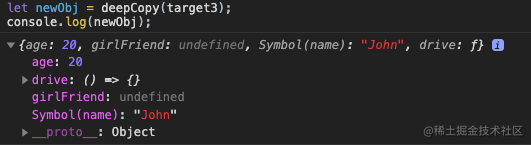### 解决循环引用

``````let target4 = {
[Symbol('name')]: 'John',
age: 20,
drive: () => {},
girlFriend: undefined
}

target4.target = target4;
``````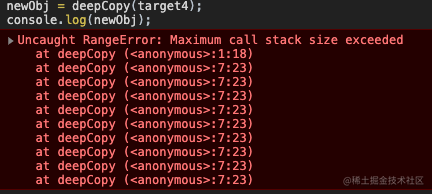``````const deepCopy2 = (originObj) => {
// 全局只能有一个记录的map，所以里面又嵌了一个方法
const map = new WeakMap();
function dp(obj){
const result = Array.isArray(obj) ? [] : {};

const existObj = map.get(obj);
// 检查map中是不是已经有这个对象了，有了就直接返回，不再递归
if(existObj){
return existObj;
}

// 没有就记录下来
map.set(obj, result);

for(let key of Reflect.ownKeys(obj)) {
if(obj.hasOwnProperty(key)){
if(obj[key] && typeof obj[key] === 'object'){
result[key] = dp(obj[key])
} else {
result[key] = obj[key];
}
}
}

return result;
}

return dp(originObj);
}
``````

`WeakMap`的兼容性不是很好，如果是老浏览器不支持`WeakMap`，我们可以用两个数组来模拟，一个数组存键，一个数组存值，每次都只在两个数组末尾新增值，这样键和值在数组中的索引就是一样的，我们可以通过这个索引来进行键和值的匹配。

## 浅拷贝的应用：mixin--混合模式

``````const mixin = {
// 注意：这里的say和run不能写成箭头函数，因为箭头函数拿不到正确的this
say() {
console.log(`\${this.name}在说话`)
},
run() {
console.log(`\${this.name}在跑步`)
}
}

class Student{
constructor(name){
this.name = name
}
}

Object.assign(Student.prototype, mixin);

const student1 = new Student('Jhon');
student1.say();
``````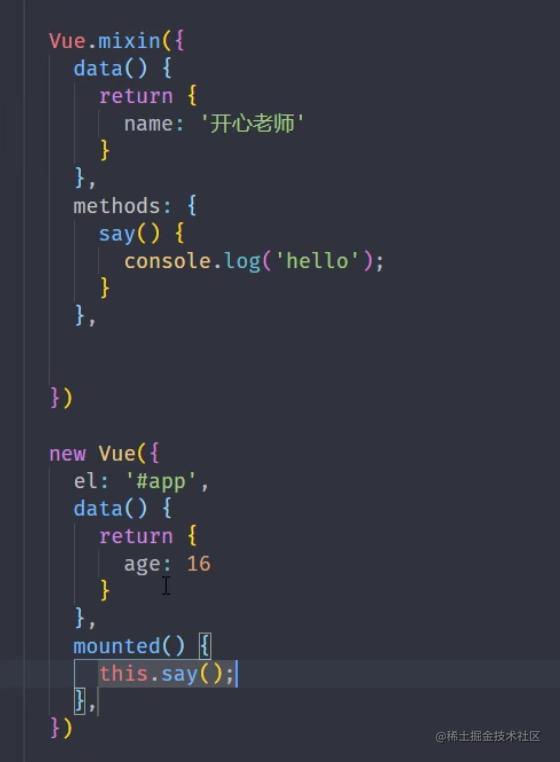## 深拷贝应用：pick函数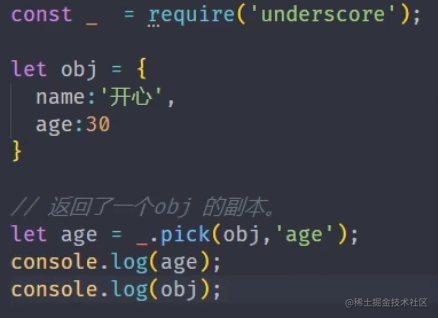``````const pick = (originObj, property) => {
const map = new WeakMap();
function dp(obj){
const result = Array.isArray(obj) ? [] : {};

const existObj = map.get(obj);

if(existObj){
return existObj;
}

map.set(obj, result);

for(let key of Reflect.ownKeys(obj)) {
// 只需要加一个检测，看看key是不是我们需要的属性就行
if(obj.hasOwnProperty(key) && key === property){
if(obj[key] && typeof obj[key] === 'object'){
result[key] = dp(obj[key])
} else {
result[key] = obj[key];
}
}
}

return result;
}

return dp(originObj);
}
``````

“前端进阶知识”系列文章：juejin.im/post/5e3ffc…

“前端进阶知识”系列文章源码GitHub地址： github.com/dennis-jian…